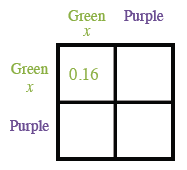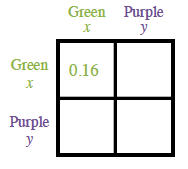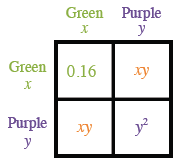### Home > GC > Chapter 5 > Lesson 5.3.2 > Problem5-83

5-83.

A particular spinner only has two regions: green and purple. If the spinner is randomly spun twice, the probability of it landing on green twice is $16\%$. What is the probability of the spinner landing on purple twice?

Find the probability of landing on green in one spin. Generic rectangle top and left sides, each labeled, green, x, & purple. Interior top left labeled, 0.16.

$x =$ the probability of landing on green in one spin.
P(green and green) $= x · x = 0.16$

$x^2 = 0.16$

$x = 0.4$

The probabilities of all possible outcomes need to add up to $1$ or $100%$%. If $x$ is $0.4$, what would $y$ be? Added to the rectangle, the label, y, underneath the purple label on top & left sides.

Use the value of $y^{^{ }2}$ to help you find the probability of landing on purple twice. Added to the interior of rectangle, top right, x, y, bottom left, x, y, bottom right, y squared.